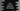# Dart continue statement explanation with example# Continue statement in dart :

continue is used to skip the current iteration of a loop. You can use continue with while, for and do-while loop. In this tutorial, we will learn how to use continue with different examples.

Normally, continue is used with a condition i.e. if this condition is true, skip the current iteration and execute the next iteration. continue can be used with nested loops as well. I am showing one example below to give you an idea of how it works.

## Continue with a while loop :

Let’s write one while loop program with continue statement :

``````main() {
var i = 0;

while (i++ < 10) {
if (i % 2 == 0) {
continue;
}
print('i = \$i');
}
}
``````

It will print the below output :

``````i = 1
i = 3
i = 5
i = 7
i = 9
``````

Here, we are printing only the odd values between 1 and 10. The loop will run from i = 1 to i = 10. Inside the loop, we have one if statement. This block checks if the current value of i is even or not. If it is even, it will execute the continue statement. That means, it will execute the next step of the while loop.

If the if else fails, i.e. if the current number is not even, it will print the current value of i.

## Continue with for loop :

continue works in a similar way with for loop. Let’s consider the below example :

``````main() {
for (var i = 0; i < 20; i++) {
if (i % 5 != 0) {
continue;
}
print('i = \$i');
}
}
``````

It will print :

``````i = 0
i = 5
i = 10
i = 15
``````

In this example, the for loop runs from i = 0 to i = 19. For each value of i, it will check if it is divisible by 5 or not. If yes, it prints its value and else it executes the continue statement i.e. executes the loop for the next value of i.

## Continue with a nested for loop :

The concept is similar. Consider the below example :

``````main() {
for (var i = 0; i < 5; i++) {
for (var j = 0; j < 5; j++) {
if (i != j) {
continue;
}
print('i = \$i, j = \$j');
}
}
}
``````

This program will print only if both i and j are equal. It will print the below output :

``````i = 0, j = 0
i = 1, j = 1
i = 2, j = 2
i = 3, j = 3
i = 4, j = 4
``````